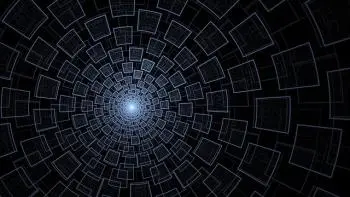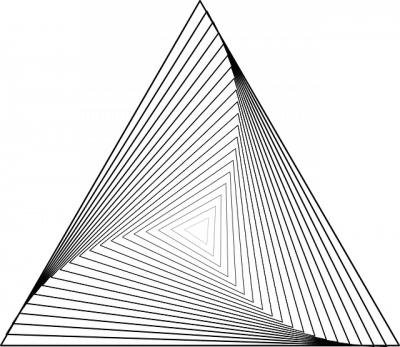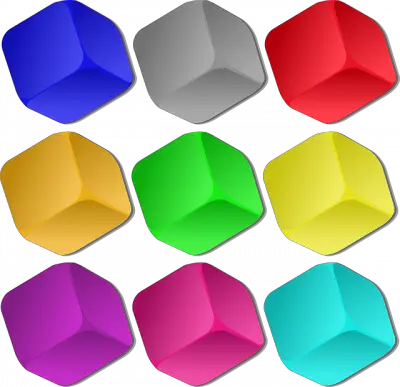# Geometry Basics: Shape and Space in 2D and 3DGeometry is a branch of mathematics that focuses on the study of the properties, relationships, and measurements of figures and objects in two- and three-dimensional space.

The basic concepts of geometry are the fundamental elements used to study geometric shapes. To enter this world it is convenient to know these basic concepts that I explain below:

## 1. Point

The point is the most basic element in geometry. It has no dimensions, that is, it has no length, width or thickness. It is represented as a simple point in space and is used to describe the location of other geometric objects.

## 2. Line

A line is an infinite collection of points that extends in both directions. It has no width or thickness, only length. Lines can be used to connect points and describe trajectories in space.

## 3. Plane

A plane is an infinite two-dimensional surface that extends in all directions. A plane is defined by at least three noncollinear points (that are not on a straight line).

Planes are fundamental in geometry to describe areas and relationships between lines and points.

## 4. Line Segment

A line segment is a finite stretch of a line that connects two points. It has a defined length and is used to measure distances between points.

## 5. Ray

A ray is a part of a line that has a starting point (origin) but extends infinitely in one direction.

Rays are used to describe paths that originate at one point and continue indefinitely in one direction.

## 6. Angle

An angle is the measure of the separation between two lines or segments that intersect at a common point, called the vertex of the angle. Angles are measured in degrees or other angular units and are used to describe relationships between lines in terms of opening and direction.

## 7. Flat Geometric ShapesA plane geometric shape is one that has two dimensions: length and width. It is limited by straight or curved lines and all its points are contained in a single plane.

Below we name some of the most notable shapes:

### Triangle

A triangle is a geometric shape made up of three line segments that connect at three vertices.

Triangles are fundamental in geometry and are classified according to their side lengths and angle measurements.

A quadrilateral is a geometric figure with four sides. Examples of quadrilaterals include squares, rectangles, and rhombuses, which have specific characteristics and properties.

### Circle

A circle is a geometric shape in which all points on the circumference are equidistant from the center. Circles have a radius (distance from the center to the circumference) and are used in numerous geometric and mathematical contexts.

### Polygon

A polygon is a geometric figure made up of line segments joined together in a closed sequence. Polygons can have any number of sides, and some common examples are triangles and quadrilaterals.

### Ellipse

An ellipse is a collection of curved lines. The sum of the distance between any point in the figure to two other fixed points is constant. The planets of the solar system orbit the Sun in elliptical orbits.

## 8. Solid Shapes

A solid shape a geometric shape that has three dimensions: height, width and length. It occupies a place in space and therefore has a volume.

Geometric bodies can be classified into two large groups: polyhedra and round bodies.

### PolyhedraPolyhedra are those in which all the delimiting surfaces are flat. Polyhedra can be classified according to the number of faces they have:

• Trihedra: they have three faces.

• Tetrahedra: they have four faces.

• Pentahedra: they have five faces.

• Hexahedrons: have six faces.

• Heptahedra: they have seven faces.

• Octahedrons: have eight faces.

• Enneahedrons: have nine faces.

• Decahedra: they have ten faces.

• Dodecahedra: they have twelve faces.

• Icosahedrons: have twenty faces.

### Round Bodies

Round bodies are those in which at least one of the delimiting surfaces is curved. The most common round bodies are:

• Sphere: it is a round body with all its parts equidistant from the center.

• Cylinder: It is a round body with two parallel circular bases and a curved side surface.

• Cone: It is a round body with a circular base and a curved lateral surface that tapers to a point called the apex.

• Ellipsoid: It is a round body with two main axes of unequal length.

• Paraboloid: It is a round body with a curved lateral surface that is a parabola.

• Hyperboloid: It is a round body with a curved lateral surface that is a hyperbola.

## 9. Perimeter

The perimeter is the sum of the lengths of the sides of a geometric figure. It is used to measure the total length of the outline of a figure, such as a square or triangle.

## 10. Area

Area is the measurement of the surface area within a geometric figure. It is expressed in square units and is used to quantify the amount of space occupied by a figure.

## 11. Volume

Volume is the measure of the space occupied by a three-dimensional object, such as a cube, sphere, or prism.

The volume of a geometric body is expressed in cubic units and is used in three-dimensional geometry.

Author:

Published: December 9, 2021
Last review: September 13, 2023